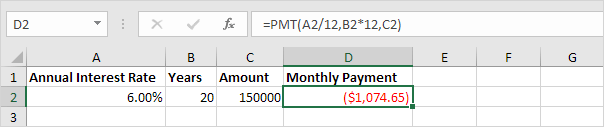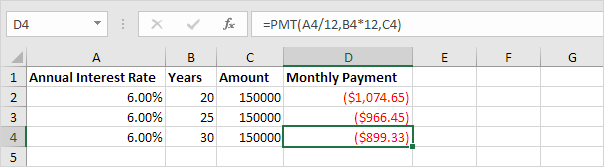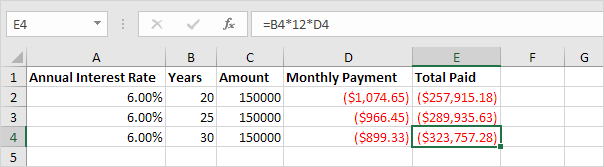# Loans with Different Durations

This example teaches you how to compare loans with different durations in Excel.

1. First, we calculate the monthly payment on a loan with an annual interest rate of 6%, a 20-year duration and a present value (amount borrowed) of \$150,000.Note: we make monthly payments, so we use 6%/12 = 0.5% for Rate and 20*12 = 240 for Nper (total number of periods).

2. Next, select the range A2:D2 and drag it down two rows.

3. Change the duration of the other two loans to 25 and 30 years.

Result:The monthly payment over 30 years (\$899,33) looks good in contrast to the \$966,45 and \$1,074.65. Right?

4. But now we calculate the Total Paid for each loan.The monthly payment over 30 years (\$899,33) suddenly does not look so attractive anymore. Conclusion: the longer the duration of the loan, the more interest you pay.

Go to Next Chapter: Statistical Functions